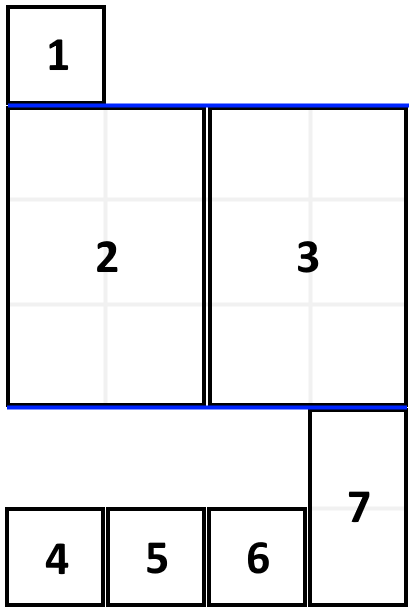1105. Filling Bookcase Shelves

We have a sequence of `books`: the `i`-th book has thickness `books[i]` and height `books[i]`.

We want to place these books in order onto bookcase shelves that have total width `shelf_width`.

We choose some of the books to place on this shelf (such that the sum of their thickness is `<= shelf_width`), then build another level of shelf of the bookcase so that the total height of the bookcase has increased by the maximum height of the books we just put down.  We repeat this process until there are no more books to place.

Note again that at each step of the above process, the order of the books we place is the same order as the given sequence of books.  For example, if we have an ordered list of 5 books, we might place the first and second book onto the first shelf, the third book on the second shelf, and the fourth and fifth book on the last shelf.

Return the minimum possible height that the total bookshelf can be after placing shelves in this manner.

Example 1:```Input: books = [[1,1],[2,3],[2,3],[1,1],[1,1],[1,1],[1,2]], shelf_width = 4
Output: 6
Explanation:
The sum of the heights of the 3 shelves are 1 + 3 + 2 = 6.
Notice that book number 2 does not have to be on the first shelf.
```

Constraints:

• `1 <= books.length <= 1000`
• `1 <= books[i] <= shelf_width <= 1000`
• `1 <= books[i] <= 1000`

1105. Filling Bookcase Shelves
``````struct Solution;

impl Solution {
fn min_height_shelves(books: Vec<Vec<i32>>, shelf_width: i32) -> i32 {
let n = books.len();
let mut dp = vec![0; n + 1];
for i in 0..n {
let mut width = books[i];
let mut height = books[i];
dp[i + 1] = height + dp[i];
for j in (0..i).rev() {
width += books[j];
if width <= shelf_width {
height = height.max(books[j]);
dp[i + 1] = dp[i + 1].min(height + dp[j]);
}
}
}
dp[n]
}
}

#[test]
fn test() {
let books = vec_vec_i32![[1, 1], [2, 3], [2, 3], [1, 1], [1, 1], [1, 1], [1, 2]];
let shelf_width = 4;
let res = 6;
assert_eq!(Solution::min_height_shelves(books, shelf_width), res);
}
``````